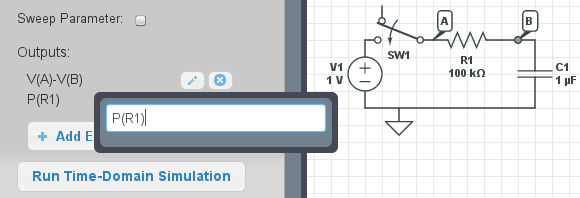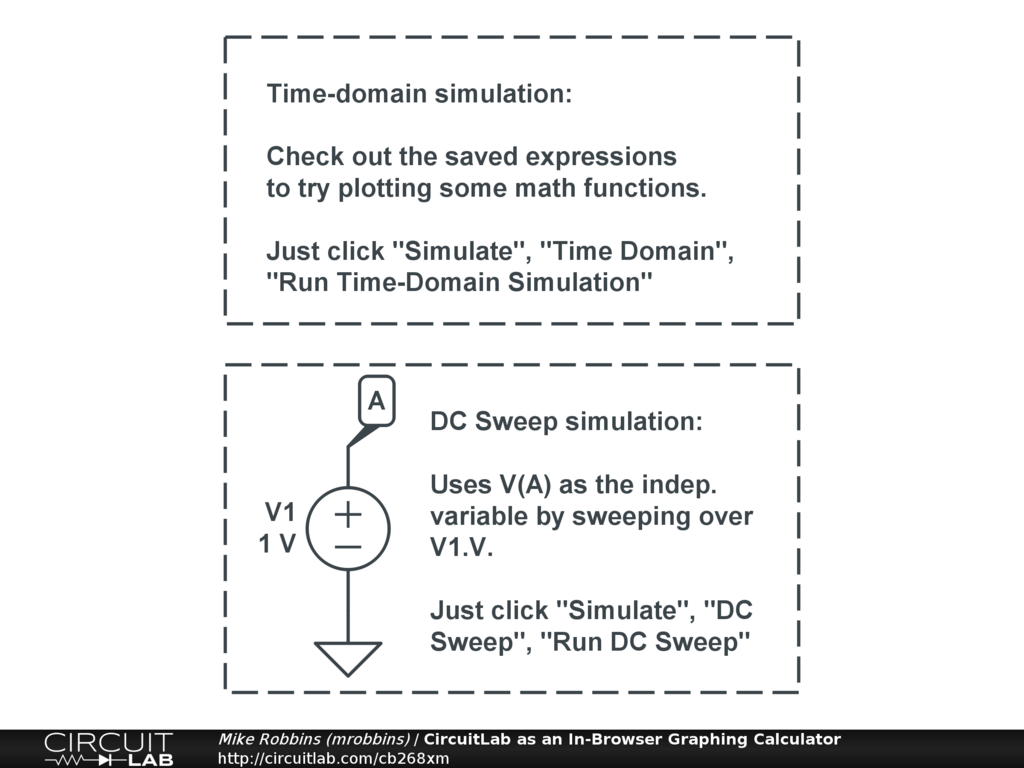## In-browser Graphing Calculator (Happy Pi Day!)

#### Mar 14 2012, 4:25 PM PDT · 0 comments »

CircuitLab's simulation and plotting engine has the ability to evaluate arbitrary mathematical expressions. That makes it easy, for example, to plot the voltage across an element, such as "V(A)-V(B)" to plot the voltage difference between those nodes.You can also try other powerful expressions, such as "P(R1)" to plot the power dissipation in element R1. This is especially useful for multi-terminal devices like transistors when you want to make sure that the power dissipation won't cause thermal problems or even device failure. Power users can also use these expressions in frequency-domain analysis, for example when evaluating the performance of differential amplifiers. Check out the documentation for more about plotting expressions in CircuitLab.

Many basic mathematical expressions can also be used when setting parameter values. For example, if we want to create an RC low-pass filter with a corner frequency of 60 Hz, and we have a resistance of 100 kOhms, we can double-click on the capacitor and enter a capacitance of "1/(2*PI*100k*60)" -- CircuitLab will compute the value automatically.Since today is Pi Day, we thought we'd show off the fact that you can actually use a tiny subset of CircuitLab to act as an in-browser graphing calculator! In time-domain simulation mode, you can use expressions with "T" to plot functions of time, so for example "T^2" to plot a simple parabola.

You can get even fancier: "-0.5*9.81*T^2 + 20*T" would show the parabolic vertical trajectory of an object launched upwards at an initial vertical velocity of 20m/s. And "2.5*COS(2*PI*T)" would make the appropriate cosine signal. DC Sweep mode can also be used to create parameters that you can adjust on both sides of zero, or even plot against a logarithmic scale.Check out this demo circuit for a few examples: